# PH

pH is a measure of the acidity and the basicity/alkalinity of a solution in terms of activity of hydrogen (H+) (strictly speaking, there is no such thing as the H+ ion; it is H3O+, called the hydroxonium ion). For dilute solutions, however, it is convenient to substitute the activity of the hydrogen ions with the concentration or molarity (mol/L) of the hydrogen ions (however, this is not necessarily accurate at higher concentrations).

## Contents

In aqueous systems, the hydrogen ion activity is dictated by the dissociation constant of water (Kw = 1.011 × 10−14 M2 at 25°C) and interactions with other ions in solution. Due to this dissociation constant, a neutral solution (hydrogen ion activity equals hydroxide ion activity) has a pH of approximately 7. Aqueous solutions with pH values lower than 7 are considered acidic, while pH values higher than 7 are considered basic.

## Etymology and definition

The concept of pH was introduced by S.P.L. Sørensen in 1909, and some sources trace it to the Latin term pondus hydrogenii. Other sources, however, attribute the name to the French term pouvoir hydrogène or puissance d'hydrogène.

Though pH is generally expressed without units, it is not an arbitrary scale; the number arises from a definition based on the activity of hydrogen ions in the solution. The pH scale is a reverse logarithmic representation of relative hydrogen ion (H+) concentration. On this scale, an upward shift by one integral number represents a ten-fold decrease in value. For example, a shift in pH from 2 to 3 represents a 10-fold decrease in H+ concentration, and a shift from 2 to 4 represents a 100-fold (10 × 10-fold) decrease in H+ concentration.

The precise formula for calculating pH is as follows:$\mbox{pH} = -\log_{10}{(a_{\mathrm{H^+}})}$

aH+ denotes the activity of H+ ions, and is dimensionless. In solutions that contain other ions, activity and concentration are not the same. The activity is an effective concentration of hydrogen ions, rather than the true concentration; it accounts for the fact that other ions surrounding the hydrogen ions will shield them and affect their ability to participate in chemical reactions. These other ions effectively change the hydrogen ion concentration in any process that involves H+.

In dilute solutions (such as tap water), the activity is approximately equal to the numeric value of the concentration of the H+ ion, denoted as [H+] (or more accurately written, [H3O+]), measured in moles per liter (also known as molarity). Therefore, it is often convenient to define pH as:$\mbox{pH} \approx -\log_{10}{\frac{[\mathrm{H^+}]}{1~\mathrm{mol/L}}} = -\log_{10} {\left|[\mathrm{H^+}]\right|}$

For both definitions, log10 denotes the base-10 logarithm; therefore, pH defines a logarithmic scale of acidity. The straight bars, indicating absolute value, make pH a dimensionless quantity. For example, if one makes a lemonade with a H+ concentration of 0.0050 moles per liter, its pH would be: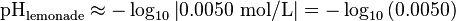$\mbox{pH}_{\mathrm{lemonade}} \approx -\log_{10}{\left|0.0050~\mathrm{mol/L}\right|} = -\log_{10}{(0.0050)}$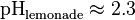$\mbox{pH}_{\mathrm{lemonade}} \approx 2.3$

A solution of pH = 8.2 will have an [H+] concentration of 10−8.2 mol/L, or about 6.31 × 10−9 mol/L. Thus, its hydrogen activity aH+ is around 6.31 × 10−9. A solution with an [H+] concentration of 4.5 × 10−4 mol/L will have a pH value of 3.35.

In solution at 25°C, a pH of 7 indicates neutrality (i.e., the pH of pure water) because water naturally dissociates into H+ and OH ions with equal concentrations of 1×10−7 mol/L. A lower pH value (for example pH 3) indicates increasing strength of acidity, and a higher pH value (for example pH 11) indicates increasing strength of basicity. Note, however, that pure water, when exposed to the atmosphere, will take in carbon dioxide, some of which reacts with water to form carbonic acid and H+, thereby lowering the pH to about 5.7.

Neutral pH at 25°C is not exactly 7. pH is an experimental value, so it has an associated error. Since the dissociation constant of water is (1.011 ± 0.005) × 10−14, the pH of water at 25°C would be 6.998 ± 0.001. The value is consistent, however, with neutral pH being 7.00 to two significant figures, which is near enough for most people to assume that it is exactly 7. The pH of water gets smaller with higher temperatures. For example, at 50°C, the pH of water is 6.55 ± 0.01. This means that a diluted solution is neutral at 50°C when its pH is around 6.55 and that a pH of 7.00 is basic.

Most substances have a pH in the range 0 to 14, although extremely acidic or extremely basic substances may have pH less than 0 or greater than 14. An example is acid mine runoff, with a pH = –3.6. Note that this does not translate to a molar concentration of 3981 M.

Arbitrarily, the pH is defined as$-\log_{10}{([\mbox{H}^+])}$. Therefore,$\mbox{pH} = -\log_{10}{[{\mbox{H}^+}]}$

or, by substitution,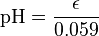$\mbox{pH} = \frac{\epsilon}{0.059}$.

The "pH" of any other substance may also be found (e.g., the potential of silver ions, or pAg+) by deriving a similar equation using the same process. These other equations for potentials will not be the same, however, as the number of moles of electrons transferred (n) will differ for the different reactions.

## Calculation of pH for weak and strong acids

Values of pH for weak and strong acids can be approximated using certain assumptions.

Under the Brønsted-Lowry theory, stronger or weaker acids are a relative concept. Here we define a strong acid as a species that is a much stronger acid than the hydronium (H3O+) ion. In that case, the dissociation reaction (strictly HX+H2O↔H3O++X but simplified as HX↔H++X) goes to completion, i.e., no unreacted acid remains in solution. Dissolving the strong acid HCl in water can therefore be expressed:

HCl(aq) → H+ + Cl

This means that in a 0.01 mol/L solution of HCl it is approximated that there is a concentration of 0.01 mol/L dissolved hydrogen ions. From above, the pH is: pH = −log10 [H+]:

pH = −log (0.01)

which equals 2.

For weak acids, the dissociation reaction does not go to completion. An equilibrium is reached between the hydrogen ions and the conjugate base. The following shows the equilibrium reaction between methanoic acid and its ions:

HCOOH(aq) ↔ H+ + HCOO

It is necessary to know the value of the equilibrium constant of the reaction for each acid in order to calculate its pH. In the context of pH, this is termed the acidity constant of the acid but is worked out in the same way (see chemical equilibrium):

Ka = [hydrogen ions][acid ions] / [acid]

For HCOOH, Ka = 1.6 × 10−4

When calculating the pH of a weak acid, it is usually assumed that the water does not provide any hydrogen ions. This simplifies the calculation, and the concentration provided by water, ×10−7 mol/L, is usually insignificant.

With a 0.1 mol/L solution of methanoic acid (HCOOH), the acidity constant is equal to:

Ka = [H+][HCOO] / [HCOOH]

Given that an unknown amount of the acid has dissociated, [HCOOH] will be reduced by this amount, while [H+] and [HCOO] will each be increased by this amount. Therefore, [HCOOH] may be replaced by 0.1 − x, and [H+] and [HCOO] may each be replaced by x, giving us the following equation:$1.6\times 10^{-4} = \frac{x^2}{0.1-x}$

Solving this for x yields 3.9×10−3, which is the concentration of hydrogen ions after dissociation. Therefore the pH is −log(3.9×10−3), or about 2.4.

## Measurement

Representative pH values
Substance pH
Hydrochloric Acid, 1M
0.1
Battery acid
0.5
Gastric acid
1.5 – 2.0
Lemon juice
2.4
Cola
2.5
Vinegar
2.9
Orange or apple juice
3.5
Beer
4.5
Acid Rain
<5.0
Coffee
5.0
Tea or healthy skin
5.5
Milk
6.5
Pure Water
7.0
Healthy human saliva
6.5 – 7.4
Blood
7.34 – 7.45
Sea water
8.0
Hand soap
9.0 – 10.0
Household ammonia
11.5
Bleach
12.5
Household lye
13.5
Caustic Soda
13.9

pH can be measured:

• By addition of a pH indicator into the solution under study. The indicator color varies depending on the pH of the solution. Using indicators, qualitative determinations can be made with universal indicators that have broad color variability over a wide pH range and quantitative determinations can be made using indicators that have strong color variability over a small pH range. Extremely precise measurements can be made over a wide pH range using indicators that have multiple equilibriums in conjunction with spectrophotometric methods to determine the relative abundance of each pH-dependent component that together make up the color of a solution, or
• By using a pH meter together with pH-selective electrodes (pH glass electrode, hydrogen electrode, quinhydrone electrode, ion sensitive field effect transistor and others).

As the pH scale is logarithmic, it doesn't start at zero. Thus the most acidic of liquids encountered can have a pH of as low as −5. The most alkaline typically has a pH of 14.

## pOH

There is also pOH, in a sense the opposite of pH, which measures the concentration of OH ions, or the basicity. Since water self-ionizes, and notating [OH] as the concentration of hydroxide ions, we have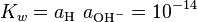$K_w = a_{{\rm{H}}^ \ } a_{{\rm{OH}}^ - }= 10^{ - 14}$ (*)

where Kw is the ionization constant of water.

Now, since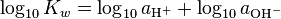$\log _{10} K_w = \log _{10} a_{{\rm{H}}^ + } + \log _{10} a_{{\rm{OH}}^ - }$

by logarithmic identities, we then have the relationship: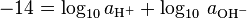$- 14 = {\rm{log}}_{{\rm{10}}} \,a_{{\rm{H}}^{\rm{ + }} } + \log _{10} \,a_{{\rm{OH}}^ - }$

and thus${\rm{pOH}} = - \log _{10} \,a_{{\rm{OH}}^ - } = 14 + \log _{10} \,a_{{\rm{H}}^ + } = 14 - {\rm{pH}}$

This formula is valid exactly for temperature = 298.15 K (25°C) only, but is acceptable for most lab calculations.

## Indicators

An indicator is used to measure the pH of a substance. Common indicators are litmus paper, phenolphthalein, methyl orange, phenol red, bromothymol blue, and bromocresol purple. To demonstrate the principle with common household materials, red cabbage, which contains the dye anthocyanin, is used.